+

Author: Sophia Tutorial
##### Description:

Simplify a square root or a cube root to determine if the result is an integer.

(more)

Sophia’s self-paced online courses are a great way to save time and money as you earn credits eligible for transfer to many different colleges and universities.*

No credit card required

37 Sophia partners guarantee credit transfer.

299 Institutions have accepted or given pre-approval for credit transfer.

* The American Council on Education's College Credit Recommendation Service (ACE Credit®) has evaluated and recommended college credit for 32 of Sophia’s online courses. Many different colleges and universities consider ACE CREDIT recommendations in determining the applicability to their course and degree programs.

Tutorial

what's covered
3. Common Squares and Cubes

An exponent is repeated multiplication. For example, 43 means that we take the base number, 4, and use it in a chain of multiplication 3 times. Notice that the exponent, 3, tells us how many times to use the base as a factor in repeated multiplication. Radicals can be used to undo this exponent operation.

Let's start with an example of a square root, since square roots are very common. A square root "unsquares" a number. For example, because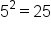, we say the square root of 25 is 5. The square root of 25 is written as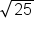.

did you know
The radical sign, when first used, was an R with a line through the tail, similar to our prescription symbol today. The R came from the Latin "Radix" which can be translated as "source" or "foundation." It wasn't until the 1500's that our current symbol was first used in Germany (but even then it was just a check mark with no bar over the numbers).

big idea

Taking the square root of a number means that we are looking for a number that, when used as a factor twice in a chain of multiplication, will give us the number underneath the radical.
What about other kinds of radicals? The cube root is also a very common radical. The cube root of a quantity is a number that is used as a factor three times in a chain of multiplication to arrive at the value underneath the radical. For example,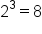because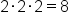. So we can say that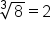, because 2 is used three times in a chain of multiplication to get 8.

This general idea applies to other types of radicals as well. We can have 4th-roots, 5th-roots, 6th-roots, and so on. Generally, we say the n-th root of a number is used in a chain of multiplication n times to arrive at the value underneath the radical symbol.

# 2. The Language of Radicals

How do we read expressions with radicals, and what do we call the different components in a radical expression? Let's take a look at an example: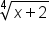This is read as "the 4th root of (x + 2)".

There are a couple of things to call out in the expression above:

1. The radical sign,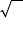, indicates that the expression is a radical expression involving a type of root.
2. The small number outside of the radical and to the left is called the index of the radical. Since the index is 4, this indicates a 4th root. If the index were 7, this would indicate a 7th root, and so on.
3. Lastly, the entire expression underneath the radical is referred to as the radicand. In the example above, (x+2) is the radicand of the radical expression.
term to know
The expression underneath a radical sign.

# 3. Common Squares and Cubes

Recognizing perfect squares and perfect cubes will be extremely useful in evaluating and simplifying radical expressions, particularly square roots and cube roots.

Perfect squares are integers squared, or raised to the power of 2. Here are some examples of perfect squares: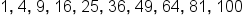The perfect squares listed above are the integers 1 through 10, squared: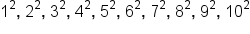Taking the square root of a perfect square results in an integer. Let's take the square root of some of the perfect squares listed above: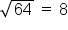because 64 is a perfect square (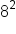).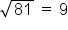because 81 is a perfect square (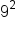).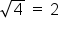because 4 is a perfect square (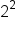).

Perfect cubes operate in a similar way, just with cube roots instead of square roots. Perfect cubes are integers cubed, or raised to the power of 3. Here are some perfect cubes: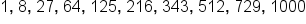The perfect cubes listed above are the integers 1 through 10 cubed: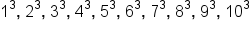Taking the cube root of a perfect cube results in an integer. Here are some examples: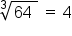because 64 is a perfect cube (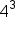)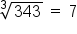because 343 is a perfect cube (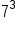)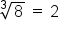because 8 is a perfect cube (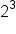)

hint

Not all square roots result in an integer. In fact, only the perfect squares do. For example,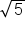is not an integer, it is an irrational number with a never-ending decimal pattern. We can approximateas 2.236. This means that 2.236 • 2.236 is about 5. If we wish to express the square root of 5 as an exact value, rather than an approximation, we leave the radical as.
summary
Radicals and exponents are inverse operations of each other. We can use the language of radicals to state the index of a radical is the number in superscript above the radical sign and the radicand is the number underneath the radical sign. Radicals can be used for any n-th root and the n-th root of a quantity is a number that is used as a factor "n" times in a chain of multiplication to arrive at the value underneath the radical. A radical expression is just an algebraic expression with the radical sign. It also helps to identify some common squares or cubes. Common squares include 4, 9, or 16, and common cubes include 8, 27, or 64.

Terms to Know